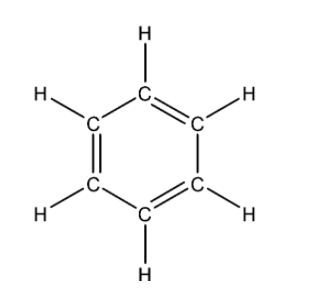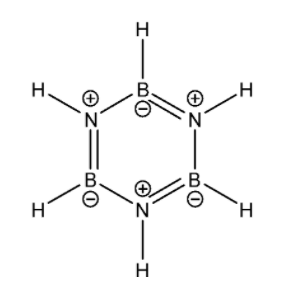QuestionAnswers

# Which of the following molecule(s)/ion(s) are isoelectronic?(a) ${ CO }_{ 2 }$ and ${ N }_{ 2 }O$(b) ${ CO }_{ 2 }$ and ${ CN }_{ 2 }^{ 2- }$(c) ${ C }_{ 6 }{ H }_{ 6 }$ and ${ B }_{ 3 }{ N }_{ 3 }{ H }_{ 6 }$(d) All are isoelectronic.

Hint: Isoelectronic species have the same number of valence electrons and similar chemical structures. For determining their chemical structure, we need to determine the hybridization of the central atoms for the respective molecules. For that we first need to determine the Lewis structures of the molecules. Always try to draw the Lewis structures such that the octet of each atom in the structure is complete.

Isoelectronic species have the same number of valence electrons and similar chemical structures. In order to draw the chemical structure of a molecule, we need to know its geometry and shape, for which we will use hybridisation and VSEPR theory. In order to determine the hybridization of an atom in a molecule we should follow the following steps:
-First you have to determine the Lewis structure of the molecule.
-Now assign the regions of electron density around the atom using VSEPR theory for predicting the shape of the molecule (single bonds, multiple bonds, radicals, and lone pairs each will count as one region).
-Now we can determine the set of hybridized orbitals corresponding to the geometry determined using VSEPR theory.
Let us start with ${ CO }_{ 2 }$ molecule. Its Lewis structure is given below:From the above structure it is clear that the regions of electron density on the central atom are two. Therefore the geometry of the molecule is linear according to VSEPR theory. For linear molecules, the central atom must undergo sp hybridization. The 2s and ${ 2p }_{ z }$ orbitals of carbon combine together in order to give two sp hybrid orbitals.
The total number of valence electrons for each atom present in the molecule is 16.
Now let us consider ${ N }_{ 2 }O$. Its Lewis structure is given below:From the above structure it is clear that the regions of electron density on the central atom are two. Therefore the geometry of the molecule is linear according to VSEPR theory. For linear molecules, the central atom must undergo sp hybridization. The 2s and ${ 2p }_{ z }$ orbitals of nitrogen atom combine together in order to give two sp hybrid orbitals.

The total number of valence electrons for each atom present in the molecule is 16.

Now let us consider ${ CN }_{ 2 }^{ 2- }$. Its Lewis structure is given below:From the above structure it is clear that the regions of electron density on the central atom are two. Therefore the geometry of the molecule is linear according to VSEPR theory. For linear molecules, the central atom must undergo sp hybridization. The 2s and ${ 2p }_{ z }$ orbitals of carbon atom combine together in order to give two sp hybrid orbitals.

The total number of valence electrons for each atom present in the molecule plus the magnitude of charge on the molecule (2) is 16.

Now let us look at ${ C }_{ 6 }{ H }_{ 6 }$. Given below is its Lewis structure:This is an aromatic organic compound and there is no one central atom. Since it is an aromatic compound, therefore it is planar, each carbon atom is ${ sp }^{ 2 }$ hybridised i.e. the geometry of the carbon atom in the molecule is Trigonal planar. Overall the molecule is planar.

The total number of valence electrons for each atom present in the molecule is 30.

Now let us look at ${ B }_{ 3 }{ N }_{ 3 }{ H }_{ 6 }$. Its Lewis structure is given below:From the Lewis structure it is clear that there is no one central atom. Around each Boron atom, there are a total of three regions of electron density. Therefore according to the VSEPR theory, its shape will be Trigonal planar and therefore it must be undergoing ${ sp }^{ 2 }$ hybridisation. Similarly around each Nitrogen atom there are three regions of electron density. Therefore it must be undergoing ${ sp }^{ 2}$ hybridisation. Overall the molecule is planar.

The total number of valence electrons for each atom present in the molecule is 30.

Hence the correct answer is (d) All are isoelectronic.

Note:
Hybridization and VSEPR theory can only be applied when the molecules contain small central atom. It cannot explain the structure of transition metal compounds since it does not concern itself with the inactive lone pairs and the sizes of the substituent groups.